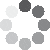Enter parameters
Enter parameters
LENGTH, WIDTH & DEPTH
Width:
ft
• inches (in)
• feet (ft)
• yards (yd)
• centimeters (cm)
• meters (m)
Length:
ft
• inches (in)
• feet (ft)
• yards (yd)
• centimeters (cm)
• meters (m)
Price per unit of area:
ft²
• square feet (ft²)
• square inches (in²)
• square yards (yd²)
• square miles (mi²)
• acres (ac)
• square centimeters (cm²)
• square meters ()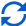Result
Total area:
0
ft²
• square feet (ft²)
• square inches (in²)
• square yards (yd²)
• square miles (mi²)
• acres (ac)
• square centimeters (cm²)
• square meters ()
Cost:
$(3 votes, average: 4.67 out of 5)Loading... Similar Calculators: Real-time graphics. Make the calculations and see the changes. Embed Similar Calculators:If you are reading this article then it is likely that you are wanting to work out how much carpet you will need when redesigning a room in your house. We’re sure you’ll agree when we say that estimating the size of a room by eye is no easy feat. You want to be as accurate as possible, saving yourself both time and money and eliminating waste. Our free online carpet calculator can help you do this. 👍 In this article we will show you just how easy it is to use and what it calculates. We will explain the mathematics behind the calculator and give some full examples. Using the carpet calculator will help you efficiently start your next home improvement project. Contents: ## Carpet calculator formula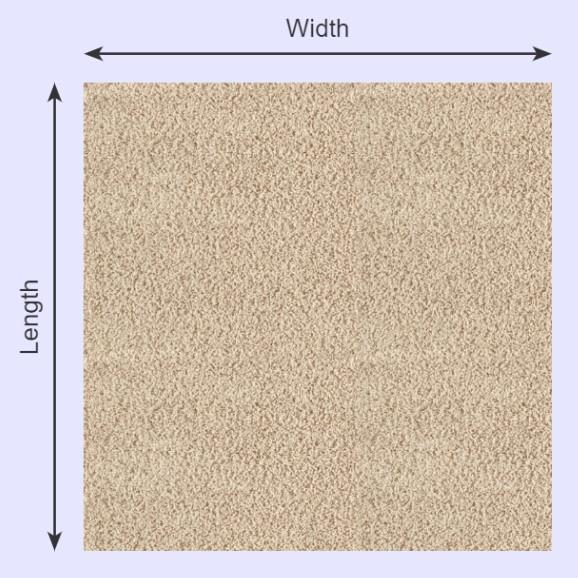In order to use the carpet calculator, you must first measure the length and width of the floor area you wish to lay down a carpet or rug. Using these measurements, the calculator will first work out the total area of the floor using the formula: $$Carpet\,Area = Length × Width$$ The standard units for length and width is feet, and for the carpet area is square footage. Later, we will show you what to do if you want to work with different units. ### Calculating the cost If you know the cost per square footage of your chosen carpet, then the calculator can work out the total cost of the home improvement project: $$Cost\,of\,Carpet = Price\,Per\,Unit\,Area × Carpet\,Area$$ It’s possible that you won’t know the cost of purchasing a square foot of the carpet. If this is the case, then you can calculate the price per unit area using the mathematical formula: $$Price\,Per\,Unit\,Area = {Total\,Cost\,of\,Carpet \over Total\,Area\,of\,Carpet}$$ At this point, it’s likely that you are wondering: ### What if I have multiple areas to carpet? In reality, we know that the room you wish to carpet is unlikely to be a perfect rectangle. It’s likely that the flooring is made up of multiple rectangles, like in our example here.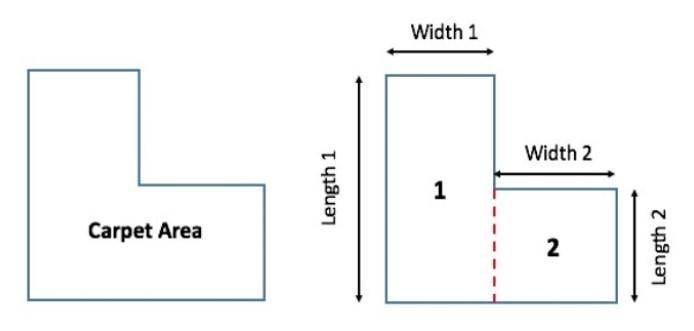Calculating the total floor area of the room you wish to lay carpet down is still very simple. 😉 Simply split the total area into individual rectangles and then calculate the area of each. Finally, find the total area of the carpet by adding up the individual areas: $$Total\,Carpet\,Area = Length\,1 × Width\,1 + Length\,2 × Width\,2 + ⋯$$ You’ll notice that the carpet calculator has an option named “add area”. You can use this to calculate the total carpet area if you have multiple rectangular areas by simply entering the values of each length and width. Confused? 😕 Keep reading to find three full examples where we start with different initial criteria. Otherwise, simply enter your measurements into the online carpet calculator to get your home improvement project off to a quick and efficient start. ## Example calculations • Carpet a singular rectangular area with known price per unit area Let’s imagine I want to cover an area with the dimensions 20 feet in length and 4 feet in width with new carpet. I can buy the carpet at a price of$5 per square foot.

I want to calculate an estimate for the amount of material I should purchase, and how much this is going to cost.

I therefore enter these values into the carpet calculator, which would perform the following operations to work out the total area of the required material:

$$Carpet\,Area = Length × Width = 20\,ft × 4\,ft = 80\,ft^2$$

In addition, it would calculate the total cost of the home improvement project:

$$Cost\,of\,Carpet = Price\,Per\,Unit\,Area × Carpet\,Area = 5\,/ft^2 × 80\,ft^2 = 400$$

• Carpet a more complicated layout

For our second example, let’s imagine we want to carpet an L-shaped room, with dimensions as shown in the picture.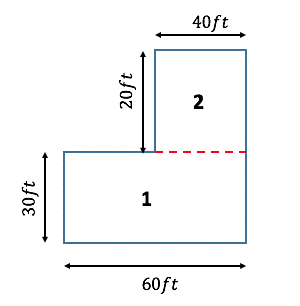I want to use new carpet that costs $7 for each square foot. Based on these measurements, the calculator is able to work out the total area of the L-shaped room in square footage: $$Total\,Carpet\,Area = Length\,1 × Width\,1 + Length\,2 × Width\,2 = 30\,ft × 60\,ft + 20\,ft × 40\,ft = 1800\,ft^2 + 800\,ft^2 = 2600\,ft^2$$ It also calculates the total cost of the project: $$Cost\,of\,Carpet = Price\,Per\,Unit\,Area × Carpet\,Area = 7\,/ft^2 × 2600\,ft^2 = 18200$$ But: What if you don’t know the price per unit of area? • Carpet a rectangular area calculating price per unit of area For our final example, let’s imagine we want to lay down a carpet in a room that measures 10 feet in length and 18 feet in length. I don’t know the price per square footage of the carpet, however I can buy 100 square feet of carpet for$750.

I can therefore calculate the price per unit of area using the formula:

$$Price\,Per\,Unit\,Area = {Total\,Cost\,of\,Carpet \over Total\,Area\,of\,Carpet} = { 750 \over 100\,ft^2} = 7.5\,/ft^2$$

At this point, I am now able to enter all the values into the online calculator to work out the total area and estimated total cost of the material:

$$Carpet\,Area = Length × Width = 10\,ft × 18\,ft = 180\,ft^2$$

$$Cost\,of\,Carpet = Price\,Per\,Unit\,Area × Carpet\,Area = 7.5\,/ft^2 × 180\,ft^2 = 1350$$

We imagine that you might be wondering:

## What if my measurements aren’t in feet and inches?

Fortunately, the answer is very easy! Our carpet calculator is able to take care of this for you.

There are multiple options for each measurement – length can be in inches (in), feet (ft), yards (yd), centimeters (cm) or meters (m).

All you need to do is to select the desired units in the options and the calculator will do the following conversions for you:

$$1\,foot = 12\,inches = 0.33\,yards = 30.48\,centimeters = 0.3048\,meters$$

$$1\,ft^2 = 144\,in^2 = 0.111\,yd^2 = 929\,cm^2= 0.0929\,m^2$$

In addition, the price can be given in dollars per square miles and square acres!

$$1\,mile^2 = 27,880,000\,ft^2$$

$$1\,acre^2 = 43,560\,ft^2$$

It’s that simple! 😉

Embed this calculator on your site!Add live graphics
Copied to clipboard! Preview